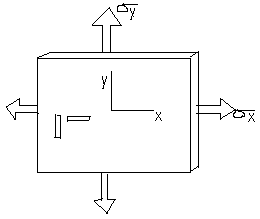# A metal alloy plate is subjected to tensile stresses of x = 8 ksi and y = 5 ksi. The strains...

## Question:

A metal alloy plate is subjected to tensile stresses of x = 8 ksi and y = 5 ksi. The strains measured in the plate are x = +969 and y = +303 . Determine the elastic modulus E for the material.

A). 6,830 ksi

B). 8,540 ksi

C). 8,120 ksi

D). 7,260 ksi

E). 6,250 ksi## Poisson's Ratio:

The value obtained after computing the division of induced lateral strain by the longitudinal strain after the application of force is known as Poisson's ratio. It is a unitless quantity and valid only in the elastic limit of the material.

Given data

• The tensile stress along x direction is: {eq}{\sigma _x} = 8\;{\rm{ksi}} {/eq}
• The tensile stress along y direction is: {eq}{\sigma _y} =...

Become a Study.com member to unlock this answer! Create your account